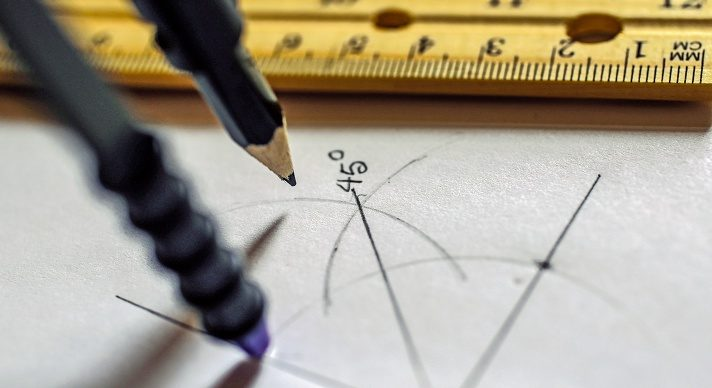# Geometry### Course Overview

Acellus Geometry provides students with a knowledge of geometric concepts and guides them through the process of developing important mathematical reasoning and proof skills. Students also gain a perspective of how geometry is an integral part of everyday life. Acellus Geometry is taught by award-winning Acellus Master Teacher, Patrick Mara. Acellus Geometry is A-G Approved through the University of California.

### Course Objectives & Student Learning Outcomes

Upon completion of this course, students will have demonstrated a mastery of geometric concepts and have developed important mathematical reasoning and proof skills. They will also be more aware of how geometry is an integral part of everyday life. Students will be familiar with parallel and perpendicular lines and how to use them to determine angle measures and congruency. Students will learn various theorems and postulates that prove triangle congruency and similarity, including SSS, SAS, ASA, and SAA Congruency Postulates and the SSS Similarity Theorem. Students know how to calculate the sum of the angles in a polygon. They also are familiar with properties of parallelograms and how to transform various geometric figures. Students have an understanding of basic relationships within triangles and have been introduced to right triangles and the basic trig functions – sine, cosine, and tangent – and have experience using them to solve problems. Students know how to calculate the area of a variety of polygons. They know how to calculate the perimeter, area, and volume of similar figures. They are experienced calculating the surface area and volume for prisms, cylinders, pyramids, cones, and spheres. Students also learn about circles. They learn how to calculate the circumference and area of circles and sectors. They are familiar with chords, arcs, and inscribed angles. Students are familiar with probability distributions and have a deeper understanding of permutations and combinations. They also know how to solve compound and conditional probability problems and have experience with probability models.This course was developed by the International Academy of Science. Learn More

### Scope and Sequence

Unit 1 – Tools of Geometry This unit covers nets and perspective drawings, points, lines, and planes.  It also covers measuring segments and angles, angle pairs, basic construction, the coordinate plane, perimeter, circumference, and area. Unit 2 – Reasoning and Proof This unit discusses inductive reasoning and conditional statements.   It also discusses biconditionals, deductive reasoning, and proofs. Unit 3 – Parallel and Perpendicular Lines This unit covers transversals, parallel lines, proving lines parallel, parallel and perpendicular, angle sum theorem, more constructions, equations of lines, and slopes of parallel and perpendicular lines. Unit 4 – Congruent Triangles This unit discusses congruent figures, SSS and SAS, ASA and SAA, and corresponding parts.  It also discusses isosceles and equilateral, right triangles, and overlapping triangles. Unit 5 – Relationships Within Triangles This unit covers midsegments, bisectors, and centers of triangles.  It also covers indirect proof, and inequalities in one and in two triangles. Unit 6 – Polygons This unit discusses polygon angle sums, parallelograms, proving a parallelogram, rhombuses, rectangles, and squares.  It also discusses proving special parallelograms, trapezoids and kites, polygons and coordinates, and using coordinates in proofs.

Following this unit students are presented with the Mid-term Review and Exam.

Unit 7 – Similarity This unit discusses ratios and proportions and similar polygons.  Also discussed are proving triangles similar, similarity in right triangles, and proportions in similar triangles. Unit 8 – Right Triangles and Trigonometry This unit discusses the Pythagorean Theorem, special right triangles, and trigonometry.  Also discussed are angles of elevation and depression, the law of sine, and the law of cosine. Unit 9 – Transformations This unit covers translations, reflections, rotations, and compositions.  Also covered are congruence transformations, dilations, and similarity transformations. Unit 10 – Area This unit discusses areas of paralelograms, triangles, trapezoids, rhombuses, kites, and regular polygons.  It also discusses circles, sectors, and perimeters and areas of similar figures. It also covers trigonometry and area, circles and arcs, lengths of arcs, area addition and subtraction, and geometric probability. Unit 11 – Surface Area and Volume This unit discusses polyhedra, and surface areas and volumes of prisms, cylinders, pyramids, spheres, and similar solids.  It also discusses volumes of cones. Unit 12 – Circles This unit covers tangent lines, chords and arcs, and inscribed angles. It also covers angle measures and segment lengths, circles in the coordinate plane, and locus. Unit 13 – Parabolas This unit explores parabolas and completing the square.  It also investigates distance, midpoint, and circles. Unit 14 – Probability This unit discusses experimental versus theoretical probability, probability distributions, permutations, combinations, compound probabilities, probability models, conditional probability, expected value, and two-way tables.

Following this unit students are presented with the Final Review and Exam.